# We Helped With This MATLAB Programming Assignment: Have A Similar One?

SOLVEDCategory Programming MATLAB Undergraduate Solved Matlab Help Online

## Assignment Description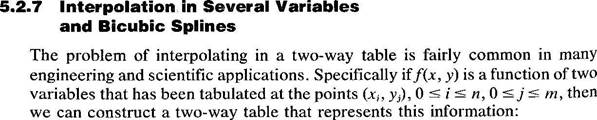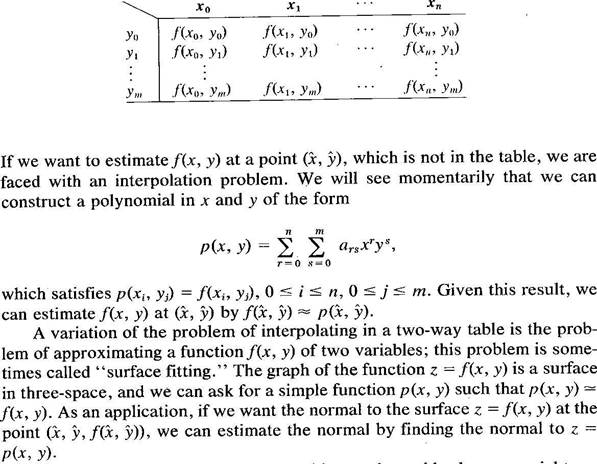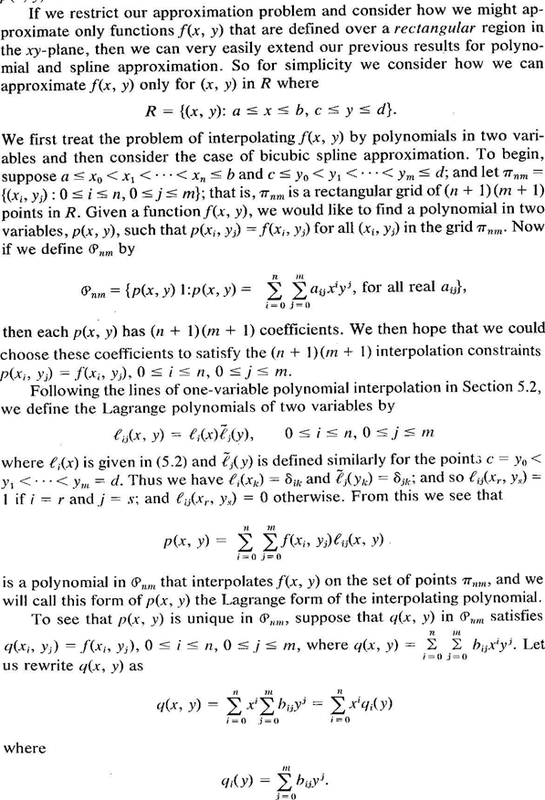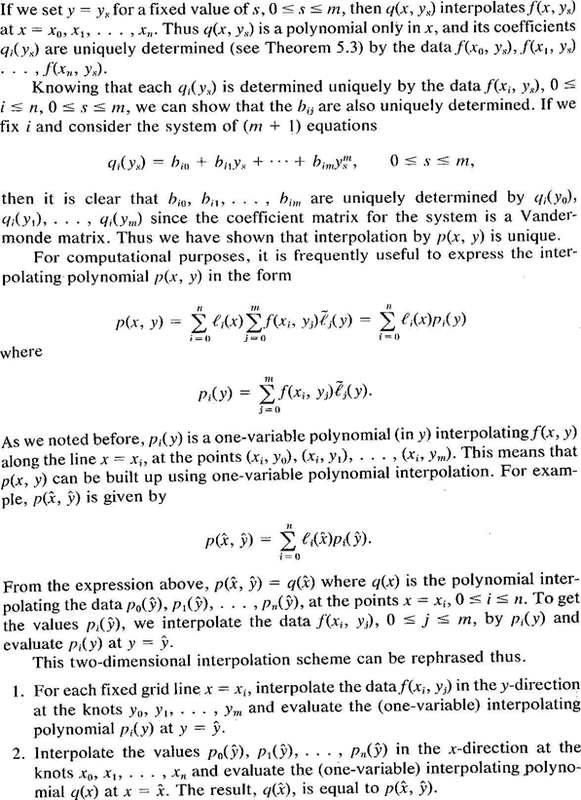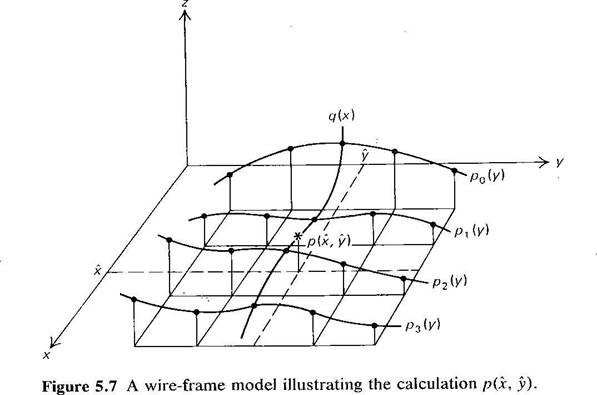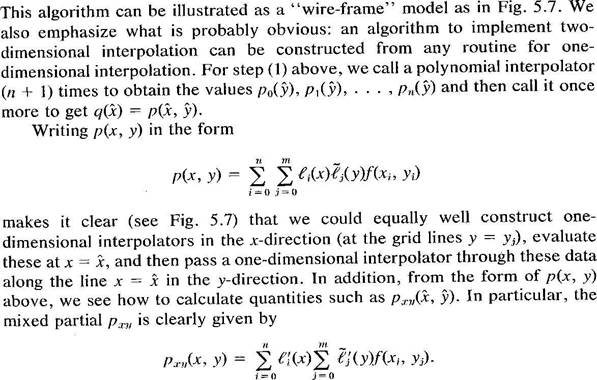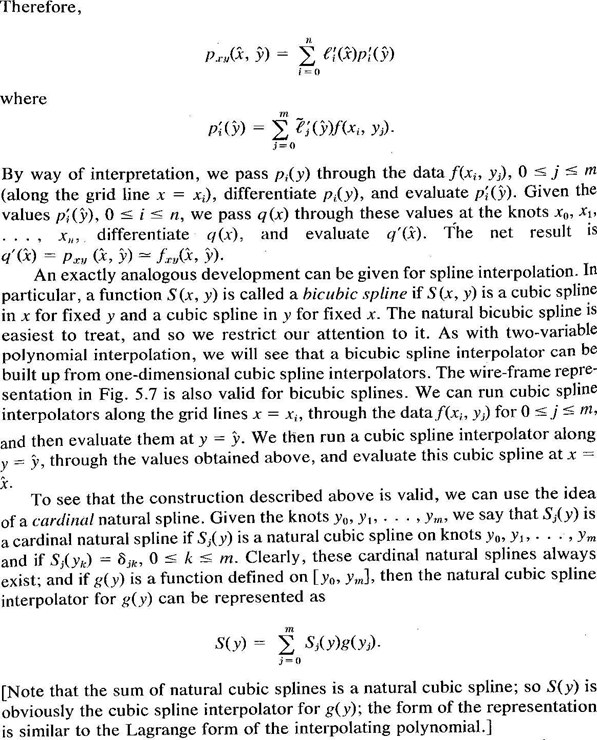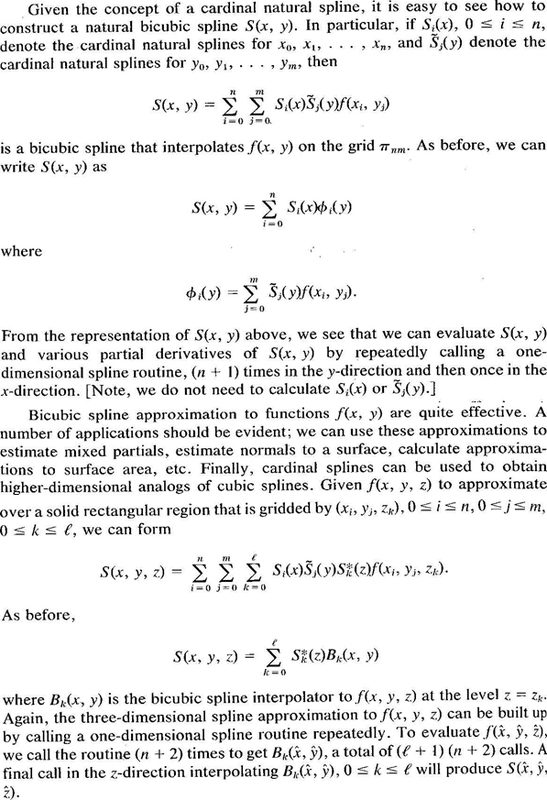## Assignment Description

CSci 5302                                        HW3                  Due: March 12, 2019

,

This homework should be done in groups of 2. Upload one copy of your answers as a single PDF file. Make sure both names are on the cover page. The resulting score will be awarded to both students.

All the computations below should be done in Matlab (except where noted).

1. Interpolate a polynomial p(t) and a natural cubic spline s(t) through the set of points (ti,yi):

 ti −2 −1 0 1 2 yi 0 1 4 1 0

.

For the interpolation polymonial use the builtin Matlab function “polyfit” as illustrated below. This function requires three arguments: the knots, the values and a third argument which must be set to the degree of the interpolating polynomial. Give the coefficients of the resulting polynomials in standard form p(t) = antn + an1tn−1 + an2tn−2 + ··· + a1t + a0.

For the cubic spline, use the Matlab function “natspline.m”. You can find this function in the “Notes” section of the class web site. Try the Matlab commands

knots = [-2 -1 0 1 2]; values = [1 1 0 1 1]; % for points y.

tt = -2:0.1:2;

p = polyfit(knots,values,4); % coefficients of interpolating polynomial yyp = polyval(p,tt);  % to evaluate the polynomial at all the points tt

pp = natspline(knots,values);% to compute the natural cubic spline

pp.coefs   % to print out the coefficients of the spline yys = ppval(pp,tt); % to evaluate the spline at many intermediate points plot(tt,yyp,’b-’,tt,yys,’r--’,knots,values,’ko’);

% plot the polynomial interpolant (blue),

% the spline (red dashed), and the knots (black circles)

legend(’interpolant’,’spline’,’knots’,’Location’,’Best’)

% the last 2 arguments optimize the placement of the legend.

Plot the resulting spline overlayed on the plot of the interpolating polynomial. The program is already given (you are using the built-in functions, except for natspline.m which can be retrieved from the class web site), so hand in just a printout of values of the coefficients of the interpolating polynomial and the spline, together with the plot. Identify which curve on the plot is the spline and which is the interpolating polynomial.

The following requires a Matlab script very similar to the above.

2. Let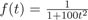. Here you will fit a polynomial and a spline to this function and then see if the error observed matches the prediction.

(a)    Using equally spaced knots knots = [-1.00, ... , 1.00], with a spacing of 1/k for each k = 2,4,6,8,10, do the following.

i.        Sample the function f at these 2k+1 knots, and let values be the corresponding f values at the knots.

ii.      Fit both an interpolating polynomial p and a natural cubic spline s through these knots.

iii.    Then sample all three curves (f, p, s) between −1 and +1 at a spacing of 1/100 (t = -1:.01:1) and find the maximum absolute differences |fp| & |fs| occuring among these sample points.

(b)    On a single plot (plot#1), show how the maximum absolute differences vary as a function of

k. Use semilogy (to draw the y-axis in a log scale) if necessary.

(c)    Also hand in a plot (plot#2) of the individual curves for k = 10. Scale the vertical axis so the original curve f is visible (by using, e.g., the axis command as shown), even if one of the approximate curves goes off the scale. The following matlab commands will draw such a plot plot(t,y,t,p,t,s,knots,values,’o’); title(’Interpolation of Runge’’s function’); xlabel(’t’);ylabel(’fcn value’) legend true interp spline knots

axis([tmin, tmax, ymin, ymax]) % to rescale the axes, if needed

where t is given above and y,s,p are the corresponding vectors of f values, s values, & p values. The axis command is used to adjust the scale of the axes. Make sure your plots and figures are clearly labeled, with titles and labelled axes. The answer to this question should include these two plots, one showing the behavior of the error as a function of the number of sample points and one showing the actual curves for k = 10. Please avoid cluttering your answers with other plots not specifically requested. If you want to include any additional material, please put it after all the answers to the entire homework, labelled as “Appendix.”

(d)    (Theoretical Analysis – please type your answer). The table below summarizes how the errorshould behave as a function of stepsize h. Ideally, as h gets smaller, the bounds suggest the maximum error should decrease, but that does not always happen. Find an explanation in terms of the error bounds below for the cases where the error increases as as h gets smaller. Are the cases where the errors decrease consistent with the error bounds?

A summary of the theoretical error bounds is as follows for n + 1 knots equally spaced in an interval [a,b] with stepsize h = (ba)/n (i.e., knots tk = a + kh, k = 0,...,n) :

 polynomial interpolation(equally spaced knots)natural or clamped cubic spline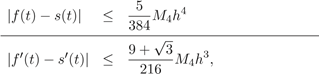all valid for t ∈ [a,b], where Mk is an upper bound on thedef k-th derivative: |f[k](t)| ≤ Mk, and W(t) = (t t0)···(t tn), so that ||W(t)||= maxt[a,b] |W(t)| ≤ hn+1n!/4.

These questions require some effort to design the code to solve them.

3. In this question, we have sampled a function at 64 points on a regular grid. The object is to fill in the intermediate values using splines, and draw the result. The values could represent either altitudes of points on a hilly terrain, or the brightness on a gray-scale image, as a function of position. You will view this in both ways.

Interpolate a 2D spline through the points on the following grid.

 y↑8 0 1 0 0 0 0 1 0 7 0 0 1 1 1 1 0 0 6 0 1 0 1 1 0 1 0 5 0 1 1 1 1 1 1 0 4 0 1 0 1 1 0 1 0 3 0 1 1 0 0 1 1 0 2 0 0 1 1 1 1 0 0 1 0 0 0 0 0 0 0 0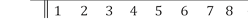We wish to evaluate this interpolating spline at all the intermediate points at a spacing of 0.02.

A detailed explanation for interpolating polynomials can be found in the “Handouts” section of the class web site under the name bicubic.pdf on the class web site. Your task is to do almost the same thing, except that you should use cubic splines instead of interpolating polynomials.

Here is a short explanation.

Using natspline.m, fit a 1D cubic spline across each row, and evaluate each spline at the intermediate points x = 1.0, 1.02, 1.04,..., 7.98, 8.0. The result will be an 8 × 351 matrix of values. Then fit a spline down each column of these intermediate values, evaluating each one at y = 1.0, 1.02, 1.04,..., 7.98, 8.0. The result will be an 351 × 351 matrix of values representing the interpolated surface across the square. Calling this matrix of values Z, plot it using the two different methods:

colormap(cool); % try different colormaps and use one you like mesh(x,y,Z);  % you should see several humps colorbar

and imagesc(Z);    % you might have to rotate the figure or use Z’ (the transpose) colormap(gray); colorbar

For the first figure, Matlab will allow you to rotate the surface so as to view it from different vantage points. The second version of the display shows a shady figure. You should hand in both figures (as first drawn) as well as a detailed description of your method. The detailed description should ideally include your code, but the code itself without sufficient comments will not be enough.

4. Paul, Jane, and Ann, share information about their likes and dislikes of movies in order to make decisions about selecting films to see. They rates films they see with a scale of 0 to 10, (10 means they liked the movie very much). Here is the status of their table of ratings when Ann was interested in a new film which soon came to a ’theater near her’ (titled ’Title 6’ in the table):

 movie Paul Jane Ann Title-1 4 8 4 Title-2 9 3 8 Title-3 2 6 1 Title-4 7 4 4 Title-5 8 3 6 Title-6 3 8 x

Ann generally follows a combination of Paul and Jane’s ratings. Ann wants to predict how well she will like the movie Title-6, which Paul and Jane have already seen. Ann reasons as follows: she will give her ’similarity’ coefficients α and β for Paul and Jane respectively. If the missing rating (call it x) were known then the column of Ann’s ratings should be the closest in the least-squares sense to the combination of Paul’s and Jane’s ratings: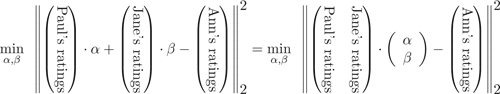Determine, α,β using the first 5 movie ratings, and form that the combination of Paul’s and Jane’s ratings that is closest to Ann’s, in a least squares sense. Use this resulting best combination to infer the induced rating for Ann for Title-6. Should Ann see ’Title 6’? Is her taste closer to Paul’s or to Jane’s?

 source: the class text and Johnson & Riess: Numerical Analysis, 2nd ed., Addison Wesley 1982.

## Assignment Description

function pp = natspline(x,y,conds)

%CSAPE Cubic spline interpolation with various end conditions.

%   Default changed to be the 'natural' cubic spline (2nd deriv's == 0 at ends)

%

%   PP  = CSAPE(X,Y)  returns the cubic spline interpolant (in ppform) to the

%   given data (X,Y) using Lagrange end conditions (the default in table below).

%   The interpolant matches, at the data site X(j), the given data value

%   Y(:,j), j=1:length(X). The data values may be scalars, vectors.

%

%   PP  = CSAPE(X,Y,CONDS)  uses the end conditions specified by CONDS, with

%   corresponding end condition values  endcondvals .

%   If there are two more data values than data sites, then the first (last)

%   data value is taken as the value for the left (right) end condition, i.e.,

%   endcondvals = Y(:,[1 end]).

%   Otherwise, default values are used.

%

%   CONDS may be a *string* whose first character matches one of the

%   following: 'complete' or 'clamped', 'periodic',

%   'second', 'variational', with the following meanings:

%

%   'complete'    : match endslopes to the slope of the cubic that

%                   matches the first four data at the respective end.

%   'not-a-knot'  : no longer supported by this function.

%   'periodic'    : match first and second derivatives at first data

%                   point with those at last data point

%                   (ignoring given end condition values if any)

%   'second'      : match end second derivatives (as given,

%                   with default [0 0], i.e., as in variational)

%   'variational' : set end second derivatives equal to zero

%                   (ignoring given end condition values if any)

%   The *default* : natural cubic spline (like 'second' w/ zero end conditions)

%

%   By giving CONDS as a 1-by-2 matrix instead, it is possible to

%   specify *different* conditions at the two endpoints, namely

%   CONDS(i) with value endcondvals(:,i), with i=1 (i=2) referring to the

%   left (right) endpoint.

%

%   CONDS(i)=j  means that the j-th derivative is being specified to

%   be endcondvals(:,i) , j=1,2.  CONDS(1)=0=CONDS(2)  means periodic end

%   conditions.

%

%   If CONDS(i) is not specified or is different from 0, 1 or 2, then

%   the default value for CONDS(i) is  1  and the default value of

%   endcondvals(:,i) is taken.  If no end condition values are specified,

%   then the default value for endcondvals(:,i) is taken to be

%

%    deriv. of cubic interpolant to nearest four points, if   CONDS(i)=1;

%                     0                                  if   CONDS(i)=2.

%

%   For example,

%

%      x = linspace(0,2*pi,9);    %% sample every 45 degrees.

%      pp = natspline( x, [1 sin(x) 0], [1 2] );

%      xx=linspace(0,2*pi,50);    %% many samples for drawing curve.

%      plot(xx,ppval(pp,xx));

%

%   gives a good approximation to the sine function on the interval [0 .. 2*pi]

%   (matching its slope 1 at the left endpoint, x(1) = 0, and its second

%   derivative 0 at the right endpoint, x(9) = 2*pi, in addition to its value

%   at every x(i), i=1:9).

%

%   The following plots a circle:

%

%      x = [0:.1:4]; pp=natspline( [0:4], [1 0 -1 0 1;0 1 0 -1 0], 'periodic');

%      y = ppval(pp,x);  plot(y(1,:),y(2,:));  axis equal;

%

%   ... Boor and The MathWorks, Inc.

%   \$Revision: 1.23 \$   editted for use in UofM class

%     Generate the cubic spline interpolant in ppform.

if nargin<3, conds = [2 2]; end

%     Generate the cubic spline interpolant in ppform.

% The fourth argument still permitted here for backward compatibility

% [xi,yi,sizeval,endvals] = chckxywp(x,y,0);

xi=x(:);

sizeval=length(x);

if sum(size(x))-1 ~= sizeval;

error('natspline: input data must be simple vectors.');

end;

if length(y) == sizeval;

yi=y(:);

endvals=[];

elseif length(y)==sizeval+2;

yi = y(2:sizeval+1);

yi=yi(:);

endvals=[y(1);y(sizeval+2)];

else

error('natspline: mismatch in vector lengths in input arguments');

end;

if ~isempty(endvals), valconds = endvals.'; end

[yn,yd] = size(yi); dd = ones(1,yd);

dx = diff(xi); divdif = diff(yi)./dx(:,dd);

[n,yd] = size(yi); dd = ones(1,yd);

valsnotgiven=0;

if ~exist('valconds','var'), valsnotgiven=1;  valconds = zeros(yd,2); end

if ischar(conds)

if     conds(1)=='c', conds = [1 1];

%elseif conds(1)=='n', conds = [1 1];

elseif conds(1)=='p', conds = [0 0];

elseif conds(1)=='s', conds = [2 2];

elseif conds(1)=='v', conds = [2 2]; valconds = zeros(yd,2);

else, error('SPLINES:CSAPE:unknownends',...

['Unknown end condition *',conds,'* specified.'])

end

end

% set up the linear system for solving for the slopes at XI.

dx = diff(xi); divdif = diff(yi)./dx(:,dd);

c = spdiags([ [dx(2:n-1,1);0;0] ...

2*[0;dx(2:n-1,1)+dx(1:n-2,1);0] ...

[0;0;dx(1:n-2,1)] ], [-1 0 1], n, n);

b = zeros(n,yd);

b(2:n-1,:)=3*(dx(2:n-1,dd).*divdif(1:n-2,:)+dx(1:n-2,dd).*divdif(2:n-1,:));

if ~any(conds)

c(1,1)=1; c(1,n)=-1;

elseif conds(1)==2

c(1,1:2)=[2 1]; b(1,:)=3*divdif(1,:)-(dx(1)/2)*valconds(:,1).';

else

c(1,1:2) = [1 0]; b(1,:) = valconds(:,1).';

if ((valsnotgiven)||(conds(1)~=1))  % if endslope was not supplied,

% get it by local interpolation

b(1,:)=divdif(1,:);

if n>2, ddf=(divdif(2,:)-divdif(1,:))/(xi(3)-xi(1));

b(1,:) = b(1,:)-ddf*dx(1); end

if n>3, ddf2=(divdif(3,:)-divdif(2,:))/(xi(4)-xi(2));

b(1,:)=b(1,:)+(ddf2-ddf)*(dx(1)*(xi(3)-xi(1)))/(xi(4)-xi(1)); end

end

end

if ~any(conds)

c(n,1:2)=dx(n-1)*[2 1]; c(n,n-1:n)= c(n,n-1:n)+dx(1)*[1 2];

b(n,:) = 3*(dx(n-1)*divdif(1,:) + dx(1)*divdif(n-1,:));

elseif conds(2)==2

c(n,n-1:n)=[1 2]; b(n,:)=3*divdif(n-1,:)+(dx(n-1)/2)*valconds(:,2).';

else

c(n,n-1:n) = [0 1]; b(n,:) = valconds(:,2).';

if ((valsnotgiven)||(conds(2)~=1))  % if endslope was not supplied,

% get it by local interpolation

b(n,:)=divdif(n-1,:);

if n>2, ddf=(divdif(n-1,:)-divdif(n-2,:))/(xi(n)-xi(n-2));

b(n,:) = b(n,:)+ddf*dx(n-1); end

if n>3, ddf2=(divdif(n-2,:)-divdif(n-3,:))/(xi(n-1)-xi(n-3));

b(n,:)=b(n,:)+(ddf-ddf2)*(dx(n-1)*(xi(n)-xi(n-2)))/(xi(n)-xi(n-3));

end

end

end

% solve for the slopes ..  (protect current spparms setting)

mmdflag = spparms('autommd');

spparms('autommd',0); % suppress pivoting

s=c;

spparms('autommd',mmdflag);

%                          .. and convert to ppform

c4 = (s(1:n-1,:)+s(2:n,:)-2*divdif(1:n-1,:))./dx(:,dd);

c3 = (divdif(1:n-1,:)-s(1:n-1,:))./dx(:,dd) - c4;

pp =  mkpp(xi.', ...

reshape([(c4./dx(:,dd)).' c3.' s(1:n-1,:).' yi(1:n-1,:).'],(n-1)*yd,4),yd);

if length(sizeval)>1, pp = fnchg(pp,'dz',sizeval); end

if ~isfield(pp,'coefs');pp.coefs=pp.P;end;

Is it free to get my assignment evaluated?

Yes. No hidden fees. You pay for the solution only, and all the explanations about how to run it are included in the price. It takes up to 24 hours to get a quote from an expert. In some cases, we can help you faster if an expert is available, but you should always order in advance to avoid the risks. You can place a new order here.

How much does it cost?

The cost depends on many factors: how far away the deadline is, how hard/big the task is, if it is code only or a report, etc. We try to give rough estimates here, but it is just for orientation (in USD):

 Regular homework \$20 - \$150 Advanced homework \$100 - \$300 Group project or a report \$200 - \$500 Mid-term or final project \$200 - \$800 Live exam help \$100 - \$300 Full thesis \$1000 - \$3000

How do I pay?

Credit card or PayPal. You don't need to create/have a Payal account in order to pay by a credit card. Paypal offers you "buyer's protection" in case of any issues.

Why do I need to pay in advance?

We have no way to request money after we send you the solution. PayPal works as a middleman, which protects you in case of any disputes, so you should feel safe paying using PayPal.

Do you do essays?

No, unless it is a data analysis essay or report. This is because essays are very personal and it is easy to see when they are written by another person. This is not the case with math and programming.

Why there are no discounts?

It is because we don't want to lie - in such services no discount can be set in advance because we set the price knowing that there is a discount. For example, if we wanted to ask for \$100, we could tell that the price is \$200 and because you are special, we can do a 50% discount. It is the way all scam websites operate. We set honest prices instead, so there is no need for fake discounts.

Do you do live tutoring?

No, it is simply not how we operate. How often do you meet a great programmer who is also a great speaker? Rarely. It is why we encourage our experts to write down explanations instead of having a live call. It is often enough to get you started - analyzing and running the solutions is a big part of learning.

What happens if I am not satisfied with the solution?

Another expert will review the task, and if your claim is reasonable - we refund the payment and often block the freelancer from our platform. Because we are so harsh with our experts - the ones working with us are very trustworthy to deliver high-quality assignment solutions on time.

Customer Feedback

"Thanks for explanations after the assignment was already completed... Emily is such a nice tutor! "

Order #13073